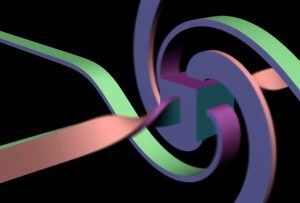# The Strange Numbers That Birthed Modern Algebra

Charlie Wood in Quanta:Imagine winding the hour hand of a clock back from 3 o’clock to noon. Mathematicians have long known how to describe this rotation as a simple multiplication: A number representing the initial position of the hour hand on the plane is multiplied by another constant number. But is a similar trick possible for describing rotations through space? Common sense says yes, but William Hamilton, one of the most prolific mathematicians of the 19th century, struggled for more than a decade to find the math for describing rotations in three dimensions. The unlikely solution led him to the third of just four number systems that abide by a close analog of standard arithmetic and helped spur the rise of modern algebra.

The real numbers form the first such number system. A sequence of numbers that can be ordered from least to greatest, the reals include all the familiar characters we learn in school, like –3.7, 5–√ and 42. Renaissance algebraists stumbled upon the second system of numbers that can be added, subtracted, multiplied and divided when they realized that solving certain equations demanded a new number, i, that didn’t fit anywhere on the real number line. They took the first steps off that line and into the “complex plane,” where misleadingly named “imaginary” numbers couple with real numbers like capital letters pair with numerals in the game of Battleship. In this planar world, “complex numbers” represent arrows that you can slide around with addition and subtraction or turn and stretch with multiplication and division.

More here.